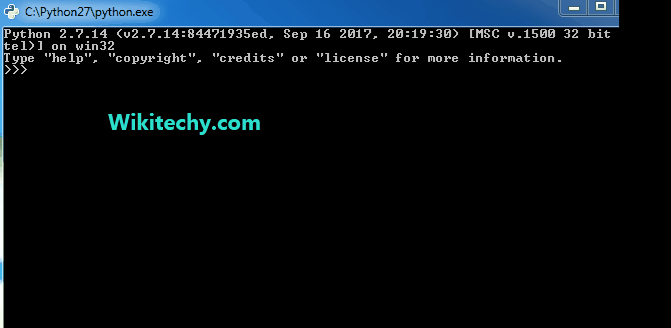# python tutorial - Python Regular Expression | Regular Expression in Python with Examples | Part 1 - learn python - python programming

Module Regular Expressions(RE) specifies a set of strings(pattern) that matches it.
To understand the RE analogy, MetaCharacters are useful, important and will be used in functions of module re.
There are a total of 14 metacharcters and will be discussed as they follow into functions:Learn Python - Python tutorial - python string regexp - Python examples - Python programs

python - Sample - python code :

• Function compile()

Regular expressions are compiled into pattern objects, which have methods for various operations such as searching for pattern matches or performing string substitutions.

python - Sample - python code :

python tutorial - Output :

Understanding the Output:
First occurrence is ‘e’ in “Aye” and not ‘A’, as it being Case Sensitive.
Next Occurrence is ‘a’ in “said”, then ‘d’ in “said”, followed by ‘b’ and ‘e’ in “Gibenson”, the Last ‘a’ matches with “Stark”.

Metacharacter blackslash ‘\’ has a very important role as it signals various sequences. If the blackslash is to be used without its special meaning as metacharcter, use’\\’

python - Sample - python code :

Set class [\s,.] will match any whitespace character, ‘,’, or,’.’ .

python - Sample - python code :

python tutorial - Output :

python - Sample - python code :

python tutorial - Output :

python - Sample - python code :

python tutorial - Output :

Understanding the Output:
Our RE is ab*, which ‘a’ accompanied by any no. of ‘b’s, starting from 0.
Output ‘ab’, is valid because of singe ‘a’ accompanied by single ‘b’.
Output ‘abb’, is valid because of singe ‘a’ accompanied by 2 ‘b’.
Output ‘a’, is valid because of singe ‘a’ accompanied by 0 ‘b’.
Output ‘abbb’, is valid because of singe ‘a’ accompanied by 3 ‘b’.

• Function split()

Split string by the occurrences of a character or a pattern, upon finding that pattern, the remaining characters from the string are returned as part of the resulting list.

Syntax :

python - Sample - python code :

The First parameter, pattern denotes the regular expression, string is the given string in which pattern will be searched for and in which splitting occurs, maxsplit if not provided is considered to be zero ‘0’, and if any nonzero value is provided, then at most that many splits occurs. If maxsplit = 1, then the string will split once only, resulting in a list of length 2. The flags are very useful and can help to shorten code, they are not necessary parameters, eg: flags = re.IGNORECASE, In this split, case will be ignored.

python - Sample - python code :

python tutorial - Output :

python - Sample - python code :

python tutorial - Output :

• Function sub()

Syntax:

python - Sample - python code :

The ‘sub’ in the function stands for SubString, a certain regular expression pattern is searched in the given string(3rd parameter), and upon finding the substring pattern is replaced by by repl(2nd parameter), count checks and maintains the number of times this occurs.

python - Sample - python code :

python - Sample - python code :

• Function subn()

Syntax:

python - Sample - python code :

subn() is similar to sub() in all ways, except in its way to providing output. It returns a tuple with count of total of replacement and the new string rather than just the string.

python - Sample - python code :

python tutorial - Output :

• Function escape()

Syntax:

python - Sample - python code :

Return string with all non-alphanumerics backslashed, this is useful if you want to match an arbitrary literal string that may have regular expression metacharacters in it.

python - Sample - python code :

python - Sample - python code :

Wikitechy tutorial site provides you all the learn python , python download , python dictionary , python snake , python list , burmese python , python 3 , python ide , python class , reticulated python , python string , python language , python online , download python , python book , python gui , python for , python threading